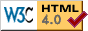## Theory Department

### Non-Renormalization Theorems in Supersymmetry

Elisabeth Kraus and Rainald Flume

In the first articles on supersymmetric field theories Wess and Zumino found that supersymmetric field theories have a better divergence structure than usual field theories. While the vanishing quadratic divergences could be immediately understood as a consequence of supersymmetry, the vanishing of radiative corrections to the superpotential was mysterious. Later, using the superspace formalism, the non-renormalization of the superpotential could be shown in general: the proof is based on explicit evaluation of supergraphs at zero momentum. Thus it was impossible to show these so-called non-renormalization theorems in the component formulation of supersymmetry or even in the Wess-Zumino gauge of gauge theories.

For the simplest supersymmetric field theory, the Wess-Zumino model, we have show that the non-renormalization theorems for the superpotential can be deduced from the cohomology structure of the supersymmetry transformations. Each invariant can be written as second supervariation of a lower dimensional field monomial and using this property one can directly establish a connection between the vertex functions and the manifestly covariant Green's functions.

Now, we proceed in investigating the various non-renormalization theorems in supersymmetry. We can now use the structurally simpler Wess-Zumino gauge and are no longer relied on the formulation in superspace where we have to worry about infrared divergences.

### Back to Theory Department or to Physics Institute.18. August 2000
www@th.physik.uni-bonn.de